# 用Python读写操作Excel数据！Stephen |25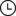2022-11-25 04:39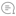0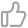00

## 2. 前期准备

### 2.1. 初识Excel

Microsoft Excel是Microsoft为使用Windows和Apple Macintosh操作系统的电脑编写的一款电子表格软件。

### 2.2. 格式区别

Excel中有xls和xlsx两种格式，它们之间的区别是：

• 文件格式不同。xls是一个特有的二进制格式，其核心结构是复合文档类型的结构，而xlsx的核心结构是XML类型的结构，采用的是基于 XML的压缩方式，使其占用的空间更小。xlsx 中最后一个 x 的意义就在于此。
• 版本不同。xls是Excel2003及以前版本生成的文件格式，而xlsx是Excel2007及以后版本生成的文件格式。
• 兼容性不同。xlsx格式是向下兼容的，可兼容xls格式。

### 2.3. 库的使用

Python自带的模块中有针对xls格式的xlrd和xlwt模块，但这两个库仅仅是针对xls的操作，当我们要操作xlsx格式文件时，则需要使用到openpyxl第三方库。

## 3. 代码展示

### 3.1. xlz格式

#### 3.1.1. 读取xls格式文件

``def read_xls_excel(url,index):    '''    读取xls格式文件    参数：        url:文件路径        index：工作表序号（第几个工作表，传入参数从1开始数）    返回：        data:表格中的数据    '''    # 打开指定的工作簿    workbook = xlrd.open_workbook(url)    # 获取工作簿中的所有表格    sheets = workbook.sheet_names()    # 获取工作簿中所有表格中的的第 index 个表格    worksheet = workbook.sheet_by_name(sheets[index-1])    # 定义列表存储表格数据    data = []    # 遍历每一行数据    for i in range(0, worksheet.nrows):        # 定义表格存储每一行数据        da = []        # 遍历每一列数据        for j in range(0, worksheet.ncols):            # 将行数据存储到da列表            da.append(worksheet.cell_value(i, j))        # 存储每一行数据        data.append(da)    # 返回数据    return data``

#### 3.1.2. 写入xls格式文件

``def write_xls_excel(url,sheet_name,two_dimensional_data):  '''    写入xls格式文件    参数：        url:文件路径        sheet_name:表名        two_dimensional_data：将要写入表格的数据（二维列表）    '''    # 创建工作簿对象    workbook = xlwt.Workbook()    # 创建工作表对象    sheet = workbook.add_sheet(sheet_name)    # 遍历每一行数据    for i in range(0,len(two_dimensional_data)):        # 遍历每一列数据        for j in range(0,len(two_dimensional_data[i])):            # 写入数据            sheet.write(i,j,two_dimensional_data[i][j])    # 保存    workbook.save(url)    print("写入成功")``

#### 3.1.3. 追加写入xls格式文件

``def write_xls_excel_add(url, two_dimensional_data, index):    '''    追加写入xls格式文件    参数：        url:文件路径        two_dimensional_data：将要写入表格的数据（二维列表）        index：指定要追加的表的序号（第几个工作表，传入参数从1开始数）    '''    # 打开指定的工作簿    workbook = xlrd.open_workbook(url)    # 获取工作簿中的所有表格    sheets = workbook.sheet_names()    # 获取指定的表    worksheet = workbook.sheet_by_name(sheets[index-1])    # 获取表格中已存在的数据的行数    rows_old = worksheet.nrows    # 将xlrd对象拷贝转化为xlwt对象    new_workbook = copy(workbook)    # 获取转化后工作簿中的第index个表格    new_worksheet = new_workbook.get_sheet(index-1)    # 遍历每一行数据    for i in range(0, len(two_dimensional_data)):        # 遍历每一列数据        for j in range(0, len(two_dimensional_data[i])):            # 追加写入数据，注意是从i+rows_old行开始写入            new_worksheet.write(i+rows_old, j, two_dimensional_data[i][j])    # 保存工作簿    new_workbook.save(url)    print("追加写入成功")``

### 3.2. xlsx格式

#### 3.2.1. 读取xlsx格式文件

``def read_xlsx_excel(url, sheet_name):    '''    读取xlsx格式文件    参数：        url:文件路径        sheet_name:表名    返回：        data:表格中的数据    '''    # 使用openpyxl加载指定路径的Excel文件并得到对应的workbook对象    workbook = openpyxl.load_workbook(url)    # 根据指定表名获取表格并得到对应的sheet对象    sheet = workbook[sheet_name]    # 定义列表存储表格数据    data = []    # 遍历表格的每一行    for row in sheet.rows:        # 定义表格存储每一行数据        da = []        # 从每一行中遍历每一个单元格        for cell in row:            # 将行数据存储到da列表            da.append(cell.value)        # 存储每一行数据        data.append(da)    # 返回数据    return data``

#### 3.2.2. 写入xlsx格式文件

``def write_xlsx_excel(url, sheet_name, two_dimensional_data):    '''    写入xlsx格式文件    参数：        url:文件路径        sheet_name:表名        two_dimensional_data：将要写入表格的数据（二维列表）    '''    # 创建工作簿对象    workbook = openpyxl.Workbook()    # 创建工作表对象    sheet = workbook.active    # 设置该工作表的名字    sheet.title = sheet_name    # 遍历表格的每一行    for i in range(0, len(two_dimensional_data)):        # 遍历表格的每一列        for j in range(0, len(two_dimensional_data[i])):            # 写入数据（注意openpyxl的行和列是从1开始的，和我们平时的认知是一样的）            sheet.cell(row=i + 1, column=j + 1, value=str(two_dimensional_data[i][j]))    # 保存到指定位置    workbook.save(url)    print("写入成功")``

#### 3.2.3. 追加写入xlsx格式文件

``def write_xlsx_excel_add(url, sheet_name, two_dimensional_data):    '''    追加写入xlsx格式文件    参数：        url:文件路径        sheet_name:表名        two_dimensional_data：将要写入表格的数据（二维列表）    '''    # 使用openpyxl加载指定路径的Excel文件并得到对应的workbook对象    workbook = openpyxl.load_workbook(url)    # 根据指定表名获取表格并得到对应的sheet对象    sheet = workbook[sheet_name]    for tdd in two_dimensional_data:        sheet.append(tdd)    # 保存到指定位置    workbook.save(url)    print("追加写入成功")``

## 4. 结果测试

### 4.1. 读取测试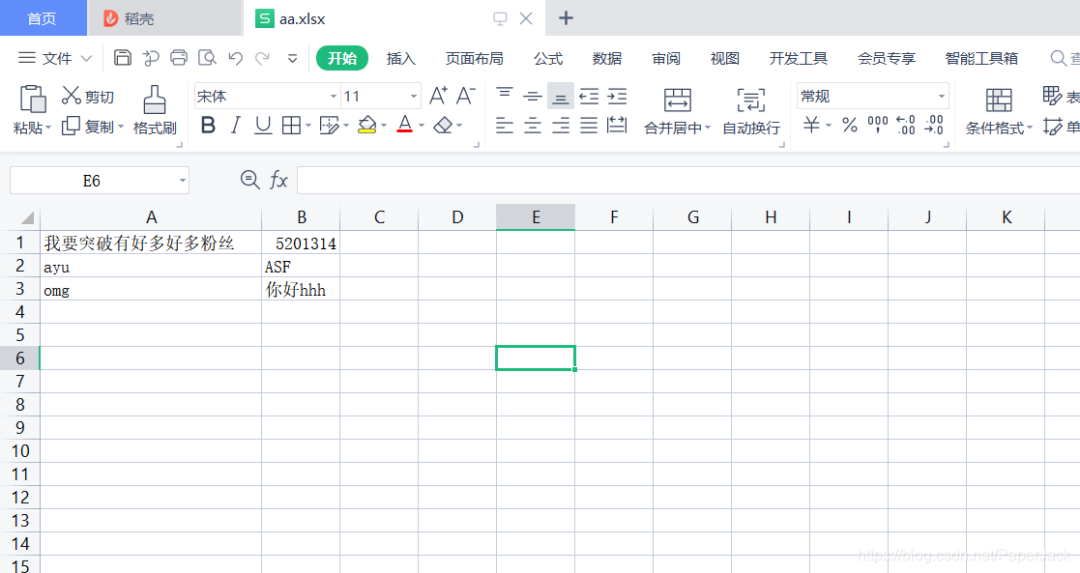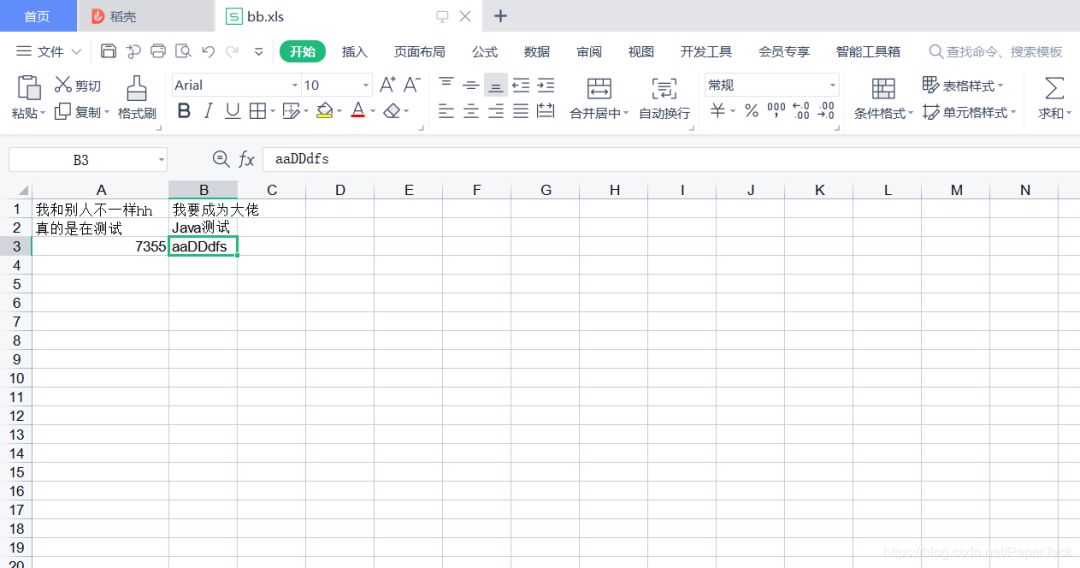## 5. 总结

END

“在看”支持下吧00

暂无评论~~
Ctrl+Enter